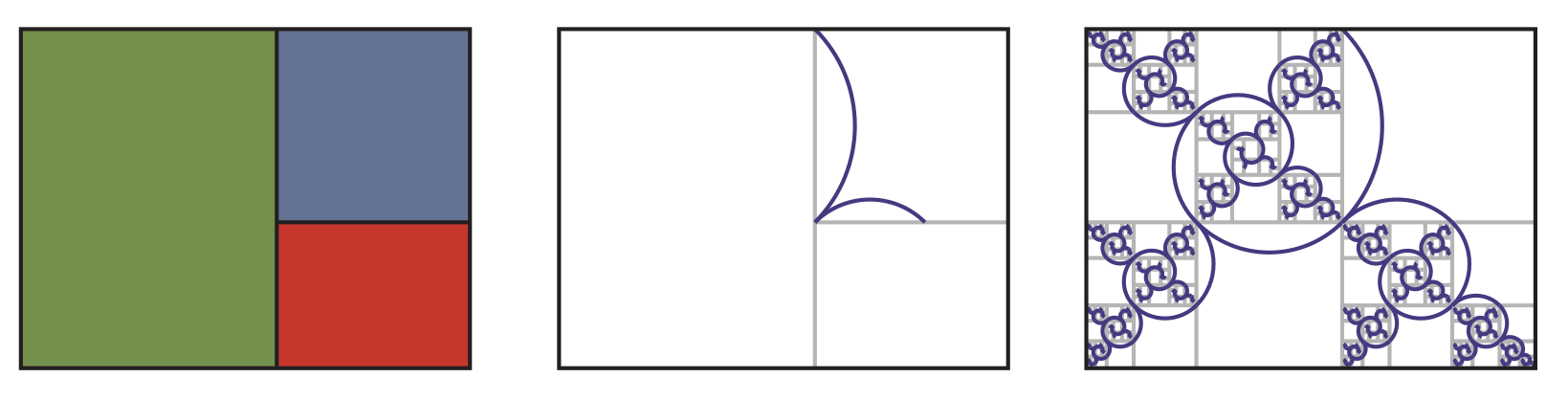# On the cover: Harriss spiral

Find out more about the spiral trees on the cover of Issue 09Image : Chalkdust.

The golden ratio (1.6180339…) has a rather overblown reputation as a mathematical path to aesthetic beauty. It is often claimed that this number is a magic constant hidden in everything from flowers to human faces. In truth, this is an exaggeration, but the number does however have some beautiful properties.

The golden ratio, often written $\phi$, is equal to $(1+\sqrt5)/2$, and is one of the solutions of the equation $x^2=x+1$. The other solution of the equation is $(1-\sqrt5)/2$, or $-1/\phi$. One of the nicest properties of the golden ratio is self-similarity: if a square is removed from a golden rectangle (a rectangle with side lengths in the golden ratio), then the remaining rectangle will also be golden. By repeatedly drawing these squares on the remaining rectangle, we can draw a golden spiral.Left: The large rectangle is golden. If a square (blue) is removed, then the remaining rectangle (green) is also golden. Right: A golden spiral. Image: Chalkdust.

Numbers that are a solution of a polynomial equation with integer coefficients are called algebraic numbers: the golden ratio is algebraic as it is a solution of $x^2=x+1$. At this point, it’s natural to wonder whether you can create interesting spirals like this with other algebraic numbers. Unsurprisingly (as otherwise, we wouldn’t be writing this article), there are other numbers that lead to pretty pictures.

The plastic ratio, $\rho=1.3247179…$, is the real solution of the equation $x\hspace{.5pt}^3=x+1$. Its exact value is
$$\rho=\sqrt{\frac{9+\sqrt{69}}{18}}+\sqrt{\frac{9-\sqrt{69}}{18}}.$$

A plastic rectangle—a rectangle with side lengths in the plastic ratio—can be split into a square and two plastic rectangles. If this splitting is repeated on the smaller plastic rectangles and two arcs are drawn in each square, a spiral is formed. These particular arcs are chosen so that they line up with the corresponding arcs drawn in the smaller rectangles.Left: The large rectangle is plastic and can be split into a square (blue) and two plastic rectangles (red) and (green). Centre: The two arcs drawn in each square. Right: A Harriss spiral.

This spiral is called the Harriss spiral, and is named after its creator Edmund Harriss. It is the shape that appears on the cover of this issue of Chalkdust, and we think its resemblance to a tree in bloom makes it perfect for spring-time. We also believe that its beauty shows that the golden ratio is a gateway into a world of mathematical creativity, not an end point. There must be other nice algebraic spirals out there, buried in the roots of polynomials. If you unearth a prize-winning specimen, let us know. You may even see it on the cover of a future issue!Matthew Scroggs is a postdoctoral researcher in the Department of Engineering at the University of Cambridge working on finite and boundary element methods. His website, mscroggs.co.uk, is full of maths.
@mscroggs    mscroggs.co.uk    + More articles by MatthewEdmund is a Clinical Assistant Professor in the Mathematics Department of the University of Arkansas, and author (with Alex Bellos) of the mathematical colouring books Snowflake Seashell Star and Visions of Numberland (called Patterns of the Universe and Visions of the Universe in the US).
@Gelada    maxwelldemon.com    + More articles by Edmund